BRAIN TEASERS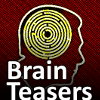# Math

These are fun math riddles. All of these tricky riddles are based on real math concepts and can be solved with purely math and logic. These are the tasks listed 1 to 10.

## Calculate the number 7983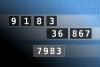NUMBERMANIA: Calculate the number 7983 using numbers [9, 1, 8, 3, 36, 867] and basic arithmetic operations (+, -, *, /). Each of the numbers can be used only once.
#brainteasers #math #numbermania

## Find number abc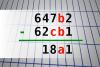If 647b2 - 62cb1 = 18a1 find number abc. Multiple solutions may exist.
#brainteasers #math

## Calculate the number 1941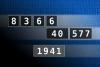NUMBERMANIA: Calculate the number 1941 using numbers [8, 3, 6, 6, 40, 577] and basic arithmetic operations (+, -, *, /). Each of the numbers can be used only once.
#brainteasers #math #numbermania### What is the sign of inflation?

What is the sign of inflation?
- A Volkswagen with 12 Latinos in it.
Jokes of the day - Daily updated jokes. New jokes every day.

## MAGIC SQUARE: Calculate A+B+C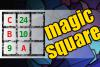The aim is to place the some numbers from the list (9, 10, 13, 14, 15, 18, 24, 25, 28, 62) into the empty squares and squares marked with A, B an C. Sum of each row and column should be equal. All the numbers of the magic square must be different. Find values for A, B, and C. Solution is A+B+C.
#brainteasers #math #magicsquare

## Find number abc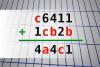If c6411 + 1cb2b = 4a4c1 find number abc. Multiple solutions may exist.
#brainteasers #math

## Calculate the number 959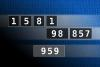NUMBERMANIA: Calculate the number 959 using numbers [1, 5, 8, 1, 98, 857] and basic arithmetic operations (+, -, *, /). Each of the numbers can be used only once.
#brainteasers #math #numbermania

## Find number abc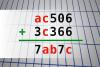If ac506 + 3c366 = 7ab7c find number abc. Multiple solutions may exist.
#brainteasers #math

## Calculate the number 4964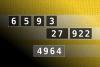NUMBERMANIA: Calculate the number 4964 using numbers [6, 5, 9, 3, 27, 922] and basic arithmetic operations (+, -, *, /). Each of the numbers can be used only once.
#brainteasers #math #numbermania

## MAGIC SQUARE: Calculate A*B+C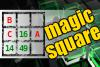The aim is to place the some numbers from the list (13, 14, 16, 20, 21, 23, 42, 49, 50, 52, 58, 79) into the empty squares and squares marked with A, B an C. Sum of each row and column should be equal. All the numbers of the magic square must be different. Find values for A, B, and C. Solution is A*B+C.
#brainteasers #math #magicsquare

## Find number abc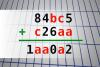If 84bc5 + c26aa = 1aa0a2 find number abc. Multiple solutions may exist.
#brainteasers #math
 NEXT PAGE
 Follow Brain Teasers on social networks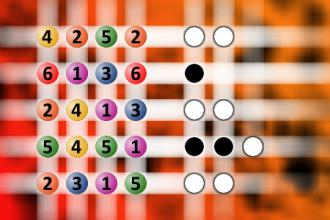The computer chose a secret code (sequence of 4 digits from 1 to 6). Your goal is to find that code. Black circles indicate the number of hits on the right spot. White circles indicate the number of hits on the wrong spot.

## Top 10 Users (1174)

 1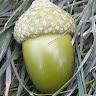H Tav 5861 2c. raj. 5777 3Nasrin 24 T 5731 4Fazil Hashim 5718 5Thinh Ddh 5557 6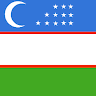Alfa Omega 4681 7Djordje Timotijevic 4549 8Mita Kojd 4525 9Jakubovski Vladimir 4443 10Chandu Rajyaguru 4419
See full ranking list

### Pierre René Deligne

Born 3 Oct 1944.Belgian mathematician who was awarded the Fields Medal at the International Congress of Mathematicians in Helsinki, Finland, in 1978 for his work in algebraic geometry. His work originated with André Weil's ideas on polynomial equations which led to three questions on what properties of a geometric object can be determined purely algebraically. These three problems quickly became major research challenges to mathematicians. A solution of the three Weil conjectures was given by Deligne. This work brought together algebraic geometry and algebraic number theory. The solution to these problems had required the development of a new kind of algebraic topology.
This site uses cookies to store information on your computer. Some are essential to help the site properly. Others give us insight into how the site is used and help us to optimize the user experience. See our privacy policy.